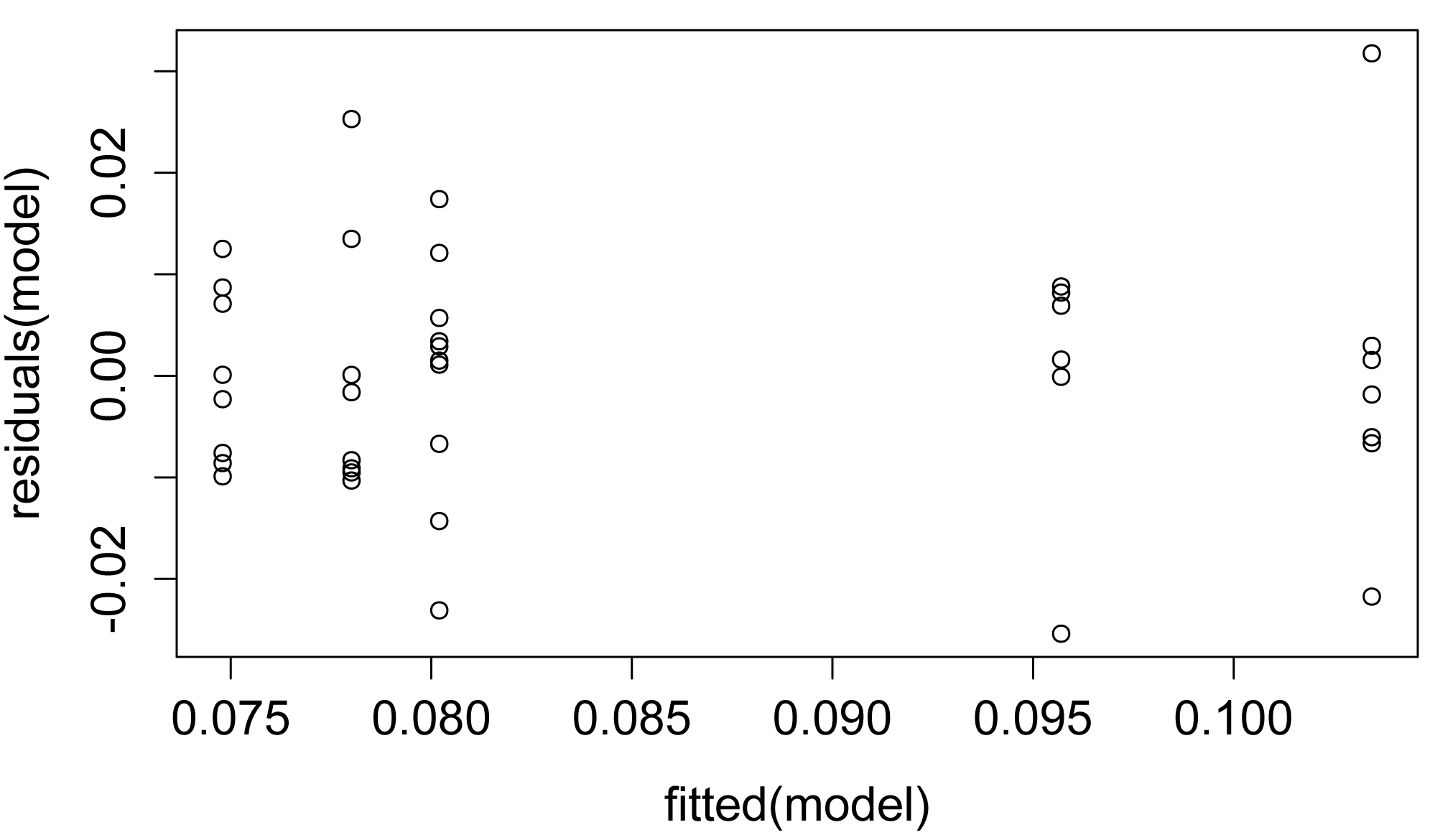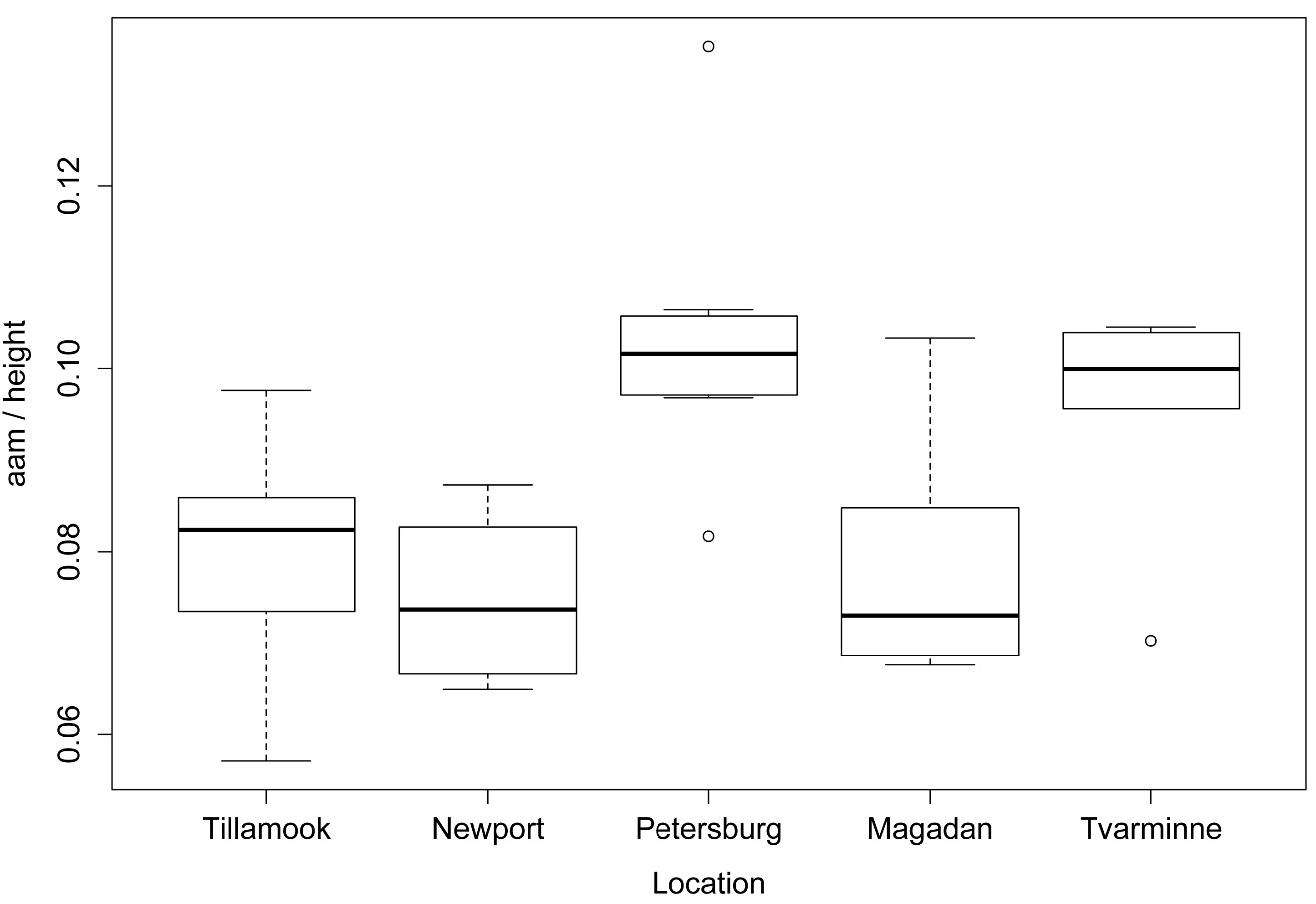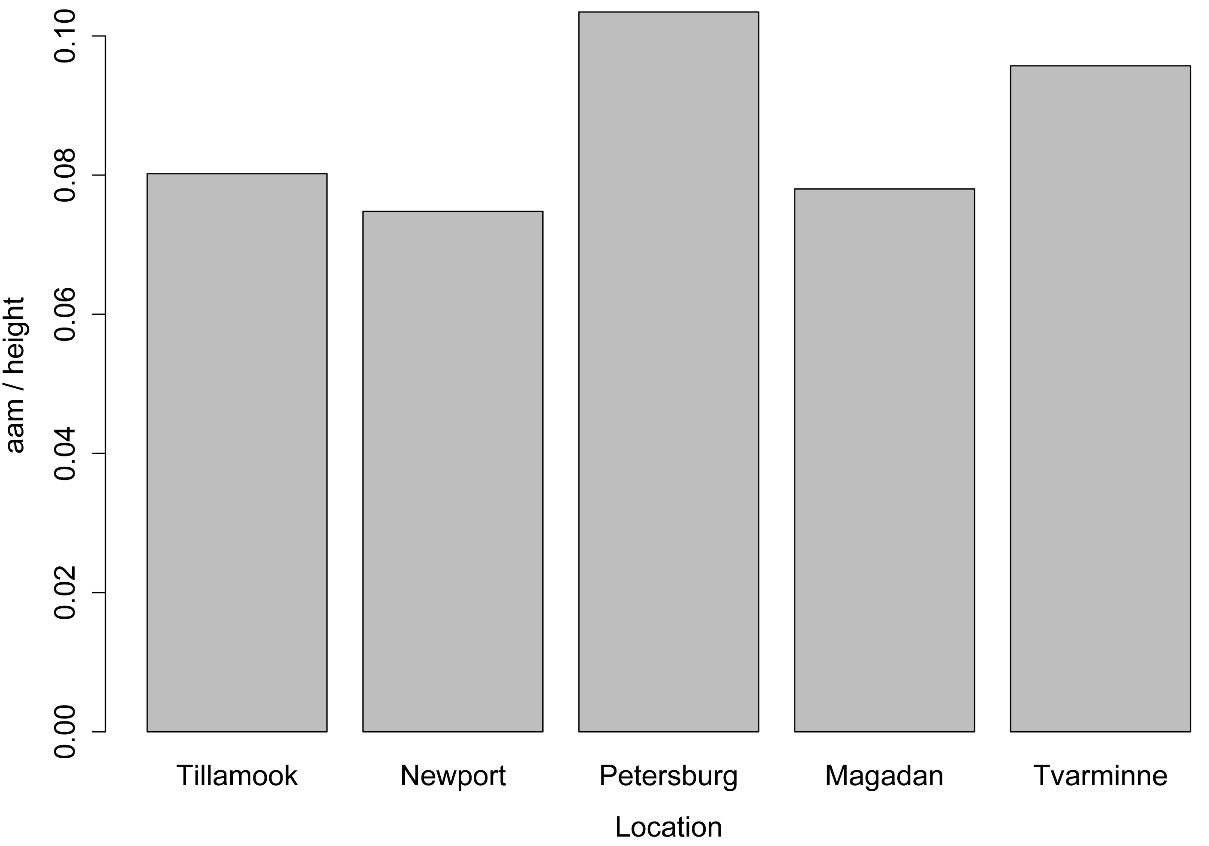## An R Companion for the Handbook of Biological Statistics

Salvatore S. Mangiafico

# One-way Anova

### Examples in Summary and Analysis of Extension Program Evaluation

SAEEPER: Introduction to Parametric Tests

### Packages used in this chapter

The following commands will install these packages if they are not already installed:

if(!require(dplyr)){install.packages("dplyr")}
if(!require(FSA)){install.packages("FSA")}
if(!require(car)){install.packages("car")}
if(!require(agricolae)){install.packages("agricolae")}
if(!require(multcomp)){install.packages("multcomp")}
if(!require(DescTools)){install.packages("DescTools")}
if(!require(lsmeans)){install.packages("lsmeans")}
if(!require(multcompView)){install.packages("multcompView")}
if(!require(Rmisc)){install.packages("Rmisc")}
if(!require(ggplot2)){install.packages("ggplot2")}
if(!require(pwr)){install.packages("pwr")}

When to use it

Analysis for this example is described below in the “How to do the test” section below.

Null hypothesis

How the test works

Assumptions

See the Handbook for information on these topics.

Tukey-Kramer test

The Tukey mean separation tests and others are shown below in the “How to do the test” section.

Partitioning variance

This topic is not covered here.

Example

Code for this example is not included here.  An example is covered below in the “How to do the test” section.

Graphing the results

Graphing of the results is shown below in the “How to do the test” section.

Similar tests

Two-sample t–test, Two-way anova, Nested anova, Welch’s anova, and Kruskal–Wallis are presented elsewhere in this book.

A permutation test, presented in the One-way Analysis with Permutation Test chapter, can also be employed as a nonparametric alternative.

### How to do the test

The lm function in the native stats package fits a linear model by least squares, and can be used for a variety of analyses such as regression, analysis of variance, and analysis of covariance.  The analysis of variance is then conducted either with the Anova function in the car package for Type II or Type III sum of squares, or with the anova function in the native stats package for Type I sum of squares.

If the analysis of variance indicates a significant effect of the independent variable, multiple comparisons among the levels of this factor can be conducted using Tukey or Least Significant Difference (LSD) procedures.  The problem of inflating the Type I Error Rate when making multiple comparisons is discussed in the Multiple Comparisons chapter in the Handbook.  R functions which make multiple comparisons usually allow for adjusting p-values.  In R, the “BH”, or “fdr”, procedure is the Benjamini–Hochberg procedure discussed in the Handbook.  See ?p.adjust for more information.

#### One-way anova example

### --------------------------------------------------------------
### One-way anova, SAS example, pp. 155-156
### --------------------------------------------------------------

Input =("
Location   Aam
Tillamook  0.0571
Tillamook  0.0813
Tillamook  0.0831
Tillamook  0.0976
Tillamook  0.0817
Tillamook  0.0859
Tillamook  0.0735
Tillamook  0.0659
Tillamook  0.0923
Tillamook  0.0836
Newport    0.0873
Newport    0.0662
Newport    0.0672
Newport    0.0819
Newport    0.0749
Newport    0.0649
Newport    0.0835
Newport    0.0725
Petersburg 0.0974
Petersburg 0.1352
Petersburg 0.0817
Petersburg 0.1016
Petersburg 0.0968
Petersburg 0.1064
Petersburg 0.1050
Tvarminne  0.0703
Tvarminne  0.1026
Tvarminne  0.0956
Tvarminne  0.0973
Tvarminne  0.1039
Tvarminne  0.1045
")

##### Specify the order of factor levels for plots and Dunnett comparison

library(dplyr)

Data =
mutate(Data,
Location = factor(Location, levels=unique(Location)))

##### Produce summary statistics

library(FSA)

Summarize(Aam ~ Location,
data=Data,
digits=3)

Location  n  mean    sd   min    Q1 median    Q3   max
1  Tillamook 10 0.080 0.012 0.057 0.075  0.082 0.085 0.098
2    Newport  8 0.075 0.009 0.065 0.067  0.074 0.082 0.087
3 Petersburg  7 0.103 0.016 0.082 0.097  0.102 0.106 0.135
4    Magadan  8 0.078 0.013 0.068 0.069  0.073 0.081 0.103
5  Tvarminne  6 0.096 0.013 0.070 0.096  0.100 0.104 0.104

##### Fit the linear model and conduct ANOVA

model = lm(Aam ~ Location,
data=Data)

library(car)

Anova(model, type="II")                    # Can use type="III"

### If you use type="III", you need the following line before the analysi
###
options(contrasts = c("contr.sum", "contr.poly"))

Sum Sq Df F value    Pr(>F)

Location  0.0045197  4   7.121 0.0002812 ***

Residuals 0.0053949 34

anova(model)                               # Produces type I sum of squares

Df    Sum Sq    Mean Sq F value    Pr(>F)

Location   4 0.0045197 0.00112992   7.121 0.0002812 ***

Residuals 34 0.0053949 0.00015867

summary(model)     # Produces r-square, overall p-value, parameter estimates

Multiple R-squared:  0.4559,  Adjusted R-squared:  0.3918

F-statistic: 7.121 on 4 and 34 DF,  p-value: 0.0002812

#### Checking assumptions of the model

hist(residuals(model),
col="darkgray")A histogram of residuals from a linear model.  The distribution of these residuals should be approximately normal.

plot(fitted(model),
residuals(model))A plot of residuals vs. predicted values.  The residuals should be unbiased and homoscedastic.  For an illustration of these properties, see this diagram by Steve Jost at DePaul University: condor.depaul.edu/sjost/it223/documents/resid-plots.gif.

### additional model checking plots with: plot(model)
### alternative: library(FSA); residPlot(model)

#### Tukey and Least Significant Difference mean separation tests (pairwise comparisons)

Tukey and other multiple comparison tests can be performed with a handful of functions.  The functions TukeyHSD, HSD.test, and LSD.test are probably not appropriate for cases where there are unbalanced data or unequal variances among levels of the factor, though TukeyHSD does make an adjustment for mildly unbalanced data.  It is my understanding that the multcomp and lsmeans packages are more appropriate for unbalanced data.  Another alternative is the DTK package that performs mean separation tests on data with unequal sample sizes and no assumption of equal variances.

##### Tukey comparisons in agricolae package

library(agricolae)

(HSD.test(model, "Location"))          # outer parentheses print result

trt     means  M
1 Petersburg 0.1034429  a
2 Tvarminne  0.0957000 ab
3 Tillamook  0.0802000 bc
5 Newport    0.0748000  c

# Means sharing the same letter are not significantly different

##### LSD comparisons in agricolae package

library(agricolae)

(LSD.test(model, "Location",   # outer parentheses print result
alpha = 0.05,

trt     means M
1 Petersburg 0.1034429 a
2 Tvarminne  0.0957000 a
3 Tillamook  0.0802000 b
5 Newport    0.0748000 b

# Means sharing the same letter are not significantly different

##### Multiple comparisons in multcomp package

Note that “Tukey” here does not mean Tukey-adjusted comparisons.  It just sets up a matrix to compare each mean to each other mean.

library(multcomp)

mc = glht(model,
mcp(Location = "Tukey"))

mcs

### Adjustment options: "none", "single-step", "Shaffer",
###                     "Westfall", "free", "holm", "hochberg",
###                     "hommel", "bonferroni", "BH", "BY", "fdr"

Linear Hypotheses:

Estimate Std. Error t value Pr(>|t|)

Newport - Tillamook == 0    -0.005400   0.005975  -0.904  0.89303

Petersburg - Tillamook == 0  0.023243   0.006208   3.744  0.00555 **

Magadan - Tillamook == 0    -0.002188   0.005975  -0.366  0.99596

Tvarminne - Tillamook == 0   0.015500   0.006505   2.383  0.14413

Petersburg - Newport == 0    0.028643   0.006519   4.394  < 0.001 ***

Magadan - Newport == 0       0.003213   0.006298   0.510  0.98573

Tvarminne - Newport == 0     0.020900   0.006803   3.072  0.03153 *

Magadan - Petersburg == 0   -0.025430   0.006519  -3.901  0.00376 **

Tvarminne - Petersburg == 0 -0.007743   0.007008  -1.105  0.80211

Tvarminne - Magadan == 0     0.017688   0.006803   2.600  0.09254 .

cld(mcs,
level=0.05,
decreasing=TRUE)

"bc"        "c"        "a"       "bc"       "ab"

### Means sharing a letter are not significantly different

##### Multiple comparisons to a control in multcomp package

### Control is the first level of the factor

library(multcomp)

mc = glht(model,
mcp(Location = "Dunnett"))

### Adjustment options: "none", "single-step", "Shaffer",
###                     "Westfall", "free", "holm", "hochberg",
###                     "hommel", "bonferroni", "BH", "BY", "fdr"

Linear Hypotheses:

Estimate Std. Error t value Pr(>|t|)

Newport - Tillamook == 0    -0.005400   0.005975  -0.904  0.79587

Petersburg - Tillamook == 0  0.023243   0.006208   3.744  0.00252 **

Magadan - Tillamook == 0    -0.002188   0.005975  -0.366  0.98989

Tvarminne - Tillamook == 0   0.015500   0.006505   2.383  0.07794 .

##### Multiple comparisons to a control with Dunnett Test

### The control group can be specified with the control option,
###   or will be the first level of the factor

library(DescTools)

DunnettTest(Aam ~ Location,
data = Data)

Dunnett's test for comparing several treatments with a control :

95% family-wise confidence level

diff       lwr.ci     upr.ci   pval

Newport-Tillamook    -0.00540000 -0.020830113 0.01003011 0.7958

Petersburg-Tillamook  0.02324286  0.007212127 0.03927359 0.0026 **

Tvarminne-Tillamook   0.01550000 -0.001298180 0.03229818 0.0778 .

##### Multiple comparisons with least square means

Least square means can be calculated for each group.  Here a Tukey adjustment is applied for multiple comparisons among group least square means.  The multiple comparisons can be displayed as a compact letter display.

library(lsmeans)
library(multcompView)

leastsquare = lsmeans(model,
pairwise ~ Location,

\$contrasts

contrast                   estimate          SE df t.ratio p.value

Tillamook - Newport     0.005400000 0.005975080 34   0.904  0.8935

Tillamook - Petersburg -0.023242857 0.006207660 34  -3.744  0.0057

Tillamook - Magadan     0.002187500 0.005975080 34   0.366  0.9960

Tillamook - Tvarminne  -0.015500000 0.006504843 34  -2.383  0.1447

Newport - Petersburg   -0.028642857 0.006519347 34  -4.394  0.0009

Newport - Magadan      -0.003212500 0.006298288 34  -0.510  0.9858

Newport - Tvarminne    -0.020900000 0.006802928 34  -3.072  0.0317

Petersburg - Magadan    0.025430357 0.006519347 34   3.901  0.0037

Petersburg - Tvarminne  0.007742857 0.007008087 34   1.105  0.8028

Magadan - Tvarminne    -0.017687500 0.006802928 34  -2.600  0.0929

P value adjustment: tukey method for comparing a family of 5 estimates

cld(leastsquare,
alpha   = 0.05,
Letters = letters,

Location      lsmean          SE df   lower.CL   upper.CL .group

Newport    0.0748000 0.004453562 34 0.06268565 0.08691435  a

Magadan    0.0780125 0.004453562 34 0.06589815 0.09012685  ab

Tillamook  0.0802000 0.003983387 34 0.06936459 0.09103541  ab

Tvarminne  0.0957000 0.005142530 34 0.08171155 0.10968845   bc

Petersburg 0.1034429 0.004761058 34 0.09049207 0.11639365    c

Confidence level used: 0.95

Conf-level adjustment: sidak method for 5 estimates

P value adjustment: tukey method for comparing a family of 5 estimates

significance level used: alpha = 0.05

#### Graphing the results

##### Simple box plots of values across groups

boxplot(Aam ~ Location,
data = Data,
ylab="aam / height",
xlab="Location")Box plots of values for each level of the independent variable for a one-way analysis of variance (ANOVA).

##### Simple bar plot of means across groups

### Summarize the data frame (Data) into a table

library(Rmisc)

Data2 = summarySE(data=Data,
"Aam",
groupvars="Location",
conf.interval = 0.95)

Tabla = as.table(Data2\$Aam)
rownames(Tabla) = Data2\$Location

Tabla

0.0802000  0.0748000  0.1034429  0.0780125  0.0957000

barplot(Tabla,
ylab="aam / height",
xlab="Location")Bar plot of means for each level of the independent variable for a one-way analysis of variance (ANOVA).

##### Bar plot of means with error bars across groups

library(ggplot2)

offset.v = -3
# offsets for mean letters
offset.h = 0.5

ggplot(Data2,
aes(x = Location, y = Aam,
ymax=0.12, ymin=0.0))  +
geom_bar(stat="identity", fill="gray50",
colour = "black", width = 0.7)  +
geom_errorbar(aes(ymax=Aam+se, ymin=Aam-se),
width=0.0, size=0.5, color="black")  +
geom_text(aes(label=c("bc","c","a","bc","ab"),
hjust=offset.h, vjust=offset.v)) +
labs(x = "Sample location",
y = "aam / height")  +

## ggtitle("Main title") +
theme_bw()  +
theme(panel.grid.major.x = element_blank(),
panel.grid.major.y = element_line(colour = "grey80"),
plot.title = element_text(size = rel(1.5),
face = "bold", vjust = 1.5),
axis.title = element_text(face = "bold"),
axis.title.y = element_text(vjust= 1.8),
axis.title.x = element_text(vjust= -0.5),
panel.border = element_rect(colour="black")
)Bar plot of means for each level of the independent variable of a one-way analysis of variance (ANOVA).  Error indicates standard error of the mean.  Bars sharing the same letter are not significantly different according to Tukey’s HSD test.

#### Welch’s anova

Bartlett’s test and Levene’s test can be used to check the homoscedasticity of groups from a one-way anova.  A significant result for these tests (p < 0.05) suggests that groups are heteroscedastic.  One approach with heteroscedastic data in a one way anova is to use the Welch correction with the oneway.test function in the native stats package.  A more versatile approach is to use the white.adjust=TRUE option in the Anova function from the car package.

### Bartlett test for homogeneity of variance

bartlett.test(Aam ~ Location,
data = Data)

Bartlett test of homogeneity of variances

Bartlett's K-squared = 2.4341, df = 4, p-value = 0.6565

### Levene test for homogeneity of variance

library(car)

leveneTest(Aam ~ Location,
data = Data)

Levene's Test for Homogeneity of Variance (center = median)

Df F value Pr(>F)

group  4    0.12 0.9744

34

### Welch’s anova for unequal variances

oneway.test(Aam ~ Location,
data=Data,
var.equal=FALSE)

One-way analysis of means (not assuming equal variances)

F = 5.6645, num df = 4.000, denom df = 15.695, p-value = 0.00508

model = lm(Aam ~ Location,
data=Data)

library(car)

Anova(model, Type="II",

Df      F   Pr(>F)

Location   4 5.4617 0.001659 **

Residuals 34

#     #     #

### Power analysis

#### Power analysis for one-way anova

### --------------------------------------------------------------
### Power analysis for anova, pp. 157
### --------------------------------------------------------------

library(pwr)

groups = 5
means = c(10, 10, 15, 15, 15)
sd = 12

grand.mean  = mean(means)
Cohen.f = sqrt( sum( (1/groups) * (means-grand.mean)^2) ) /sd

pwr.anova.test(k = groups,
n = NULL,
f = Cohen.f,
sig.level = 0.05,
power = 0.80)

Balanced one-way analysis of variance power calculation

n = 58.24599

NOTE: n is number in each group

#     #     #